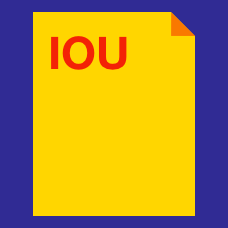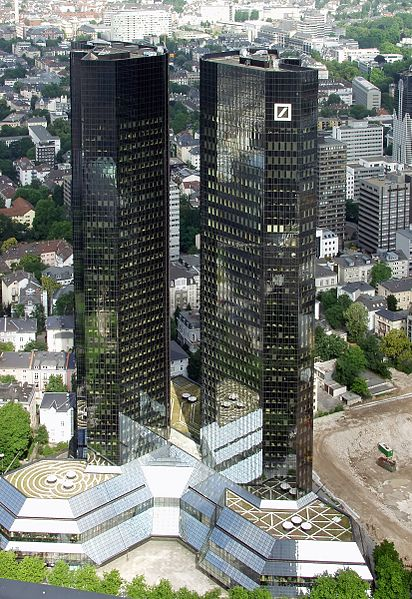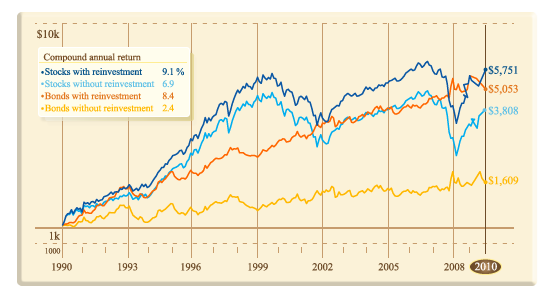Quantitative Finance

# Bond Pricing: Level 1 Challenges

Find the single percentage discount which is equivalent to a discount of 20% followed by a 30% discount.Approximately how much do you have to place in a bank which pays 1% interest per annum (compounded annually), so that you will have $100 in it in 10 years? ###### Image credit: Wikipedia ©Raimond Spekking / CC BY-SA 4.0 You own a bond that will pay 10% per year for the next 10 years, on a principal of$1000. The prevailing discount rate is 10% throughout.

What is the bond worth now?

The discount rate is the interest rate that is used in discounted cash flow analysis.

A 5 year, 5% coupon $1000 corporate bond yielding 5% is currently trading for$995. What trade would you want to execute?

Note: Ignore transaction costs and interest rates.You want to value stock dividends that are projected to grow forever at a constant rate $g$, using a discount rate of $r$. If the dividend in the first period is $D$, what is the value of this cash flow?

Assume that $r > g$.

×